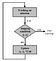# The Math Behind SVM

part3: The Implementation

Let’s start, In this tutorial we would be building SVM classifier from scratch. We would be using python scripting language along with libraries for numeric calculations and visualization. This tutorial can be divided into 3 steps:

• Step1: Build dataset
• Step2: Train SVM, ie. defining fit method & Optimizing quadratic programming problem.
• Step3: Prediction

# 1. The Dataset

Let’s import libraries, and prepare linearly non-separable dataset for model

# 2. Train SVM

## a. Initialize SVM parameters:

C:= float, default=1.0 {Regularization parameter }

kernel{‘linear’, ‘poly’, ‘rbf’, ‘sigmoid’, ‘precomputed’}

degree: degree of polynomial kernel function

gamma{‘scale’, ‘auto’} or float, default=’scale’

tol :float, default=1e-3

max_iter: int default=-1

## b. Defining fit method of SVM

The training of SVMs is characterized by convex optimization problem; thus local minima can be avoided by using polynomial-complexity Quadratic Programming (QP) methods.

Let’s minimize this constrained using optimisation problem Lagrange multipliers are used to solve it!SVM training steps

The basic algorithm for SVM training involves three main procedures (Fig.1) :

1.)the selection of the working set

2.) the verification of stopping criteria, and

3) the update of the crucial quantities, namely, αi , αj , and ∇f(α).

The first two steps clearly play a crucial role to the ultimate effectiveness of the training algorithm.

Working set selection is an important step in decomposition methods for training support vector machines (SVMs). Let’s code for technique of working set selection in SMO-type decomposition methods.

Let’s extract support vectors(An important consequence of this geometric description is that the max-margin hyperplane is completely determined by those x that lie nearest to it. These xi are called support vectors.)

for alpha, where yi(w⋅x⋆+b)−1 is zero. These examples are called support vectors, which are the closest points to the hyperplane. In the dual coefficients, they are ordered according to the class they belong to. The Blue dots in the figure below shows Support vectors!

# 4. Results:

If you are getting this far, congratulations! Thanks for reading, we hope you liked our series of Implementing SVM from scratch!! Check out the references listed below if you want to master the underlying logic of an algorithm.

References::

1. https://leon.bottou.org/publications/pdf/lin-2006.pdf

Check out our website! https://eliteaihub.com/

we keep on adding blogs, tools and videos to help to understand the math behind ML and AI !!

Helping you build something Innovative…

## More from EliteAI

Helping you build something Innovative…